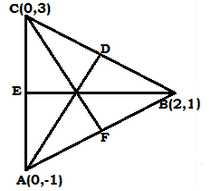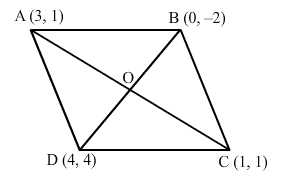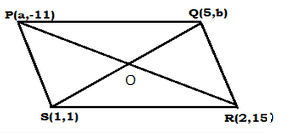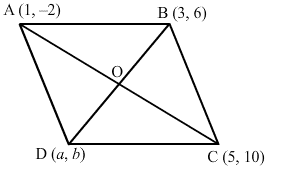RS Aggarwal Class 10 Solutions Chapter 16 - Coordinate Geometry Ex 16D (16.4)

RS Aggarwal Class 10 Chapter 16 - Coordinate Geometry Ex 16D (16.4) Solutions Free PDF

Q1) Find the ratio in which the point P(m,6) divides the join of A(-4,3) and B(2,8). Also, find the value of m.

Let the point P(m, 6) divide the line AB in the ratio k : 1.

Then, by the section formula:

x = $\frac{\left(mx_{2}+nx_{1}\right )}{\left(m+n \right )}$ , y = $\frac{\left(my_{2}+ny_{1}\right )}{\left(m+n \right )}$

The coordinates of P are (m, 6).

m = $\frac{2k-4}{k+1}$ , 6 = $\frac{8k+3}{k+1}$

$\Rightarrow$ m (k+1) = 2k – 4 , 6k + 6 = 8k + 3

$\Rightarrow$ m (k+1) = 2k – 4 , 6 – 3 = 8k – 6k

$\Rightarrow$ m (k+1) = 2k – 4 , 2k = 3

$\Rightarrow$ m (k+1) = 2k – 4 , k = $\frac{3}{2}$

Therefore, the point P divides the line AB in the ratio 3 : 2.

Now, putting the value of k in the equation m (k + 1) = 2k – 4 , we get

m($\frac{3}{2}+1$) = 2($\frac{3}{2}$) – 4

$\Rightarrow$m ($\frac{3}{2}+1$) = 3 – 4

$\Rightarrow$ $\frac{5m}{2}+1$ = -1

$\Rightarrow$ 5m = -2

$\Rightarrow$ m = $\frac{-2}{5}$

Therefore, the value of m = $\frac{-2}{5}$

So, the coordinates of P are ($\frac{-2}{5},6$)

Q2) Find the ratio in which the point (-3,k) divides the join of A(-5,-4) and B(-2,3). Also, find the value of k.

Let the point P(-3, k) divide the line AB in the ratio s : 1.

Then, by the section formula:

x = $\frac{\left(mx_{2}+nx_{1}\right )}{\left(m+n \right )}$ , y = $\frac{\left(my_{2}+ny_{1}\right )}{\left(m+n \right )}$

The coordinates of P are (-3, k)

-3 = $\frac{-2s-5}{s+1}$ , k = $\frac{3s-4}{s+1}$

$\Rightarrow$-3s-3 = -2s – 5, k(s+1) = 3s – 4

$\Rightarrow$ -3s+2s = -5+3, k(s+1) = 3s – 4

$\Rightarrow$ – s = – 2, k(s+1) = 3s – 4

$\Rightarrow$ s = 2, k(s+1) = 3s – 4

Therefore, the point P divides the line AB in the ratio 2 : 1.

Now, putting the value of k in the equation k (s + 1) = 3s – 4, we get

k(2+1) = 3(2) – 4

$\Rightarrow$ 3k = 6-4

$\Rightarrow$ 3k = 2

Therefore, the value of k = $\frac{2}{3}$

So, the coordinates of P are ($-3,\frac{2}{3}$)

Q3) In what ratio is the line segment joining A(2,-3) and B(5,6) divided by the X-Axis? Also, find the co-ordinates of the point of division.

Let AB be divided by the x-axis in the ratio k : 1 at the point P

Then, by the section formula the co-ordinates of P are

x = $\frac{\left(mx_{2}+nx_{1}\right )}{\left(m+n \right )}$ , y = $\frac{\left(my_{2}+ny_{1}\right )}{\left(m+n \right )}$

P = ( $\frac{5k+2}{k+1}, \; \frac{6k-3}{k+1}$ )

But P lies on the x-axis, so its ordinate is 0

Therefore, $\frac{6k-3}{k+1}$ = 0

$\Rightarrow$ 6k- 3 = 0

$\Rightarrow$ 6k = 3

$\Rightarrow$ k = $\frac{3}{6}$

$\Rightarrow$ k = $\frac{1}{2}$

Therefore, the required ratio is $\frac{1}{2}:1$, which is same as 1:2

Thus, the x-axis divides the line AB in the ratio is 1:2 at the point P.

Applying k = $\frac{1}{2}$, we get the co-ordinates of point:

P($\frac{5k+2}{k+1},0$)

= $P\left (\frac{5*\frac{1}{2}+2}{\frac{1}{2}+1},0\right)$

= $P\left (\frac{\frac{5+4}{2}}{\frac{1+2}{2}},0\right )$

= P$\left (\frac{9}{3},0\right )$

= P(3,0)

Hence, the point of intersection of AB and the x-axis is P(3,0)

Q4) In what ratio is the line segment joining the points A(-2, -3) and B(3,7) divided by the Y-axis ? Also, find the co-ordinates of the point of division.

Let AB be divided by the x-axis in the ratio k : 1 at the point P.

Then, by section formula the coordinates of P are

x = $\frac{\left(mx_{2}+nx_{1}\right )}{\left(m+n \right )}$ , y = $\frac{\left(my_{2}+ny_{1}\right )}{\left(m+n \right )}$

P = ( $\frac{3k-2}{k+1} ,\; \frac{7k-3}{k+1}$ )

But P lies on the y-axis; so, its abscissa is 0.

Therefore, $\frac{3k-2}{k+1}$ = 0

$\Rightarrow$ 3k- 2 = 0

$\Rightarrow$ 3k = 2

$\Rightarrow$ k = $\frac{2}{3}$

$\Rightarrow$ k = $\frac{2}{3}$

Therefore, the required ratio is $\frac{2}{3}:1$ which is same as 2 : 3

Thus, the x-axis divides the line AB in the ratio 2:3 at the point P.

Applying k = $\frac{2}{3}$,we get the coordinates of point P.

P($0,\frac{7k-3}{k+1}$)

= $P\left (0,\frac{7*\frac{2}{3}-3}{\frac{2}{3}+1}\right)$

= $P\left (0,\frac{\frac{14-9}{3}}{\frac{2+3}{3}},0\right )$

= P$\left (0,\frac{5}{5}\right )$

= P(0,1)

Hence, the point of intersection of AB and the x-axis is P(0,1)

Q5) In what ratio does the line x-y-2 = 0 divide the line segment joining the points A(3, -1) and B(8,9) ?

Let the line x – y – 2 = 0 divide the line segment joining the points A(3,-1) and B(8,9) in the ratio k:1 at P

Then, by section formula the coordinates of P are

x = $\frac{\left(mx_{2}+nx_{1}\right )}{\left(m+n \right )}$ , y = $\frac{\left(my_{2}+ny_{1}\right )}{\left(m+n \right )}$

P = ($\frac{8k+3}{k+1}) – (\frac{9k-1}{k+1}$)

Since, P lies on the line x – y – 2 = 0, we have.

$(\frac{8k+3}{k+1})-( \frac{9k-1}{k+1})-2 = 0$

$\Rightarrow$ 8k + 3 – 9k + 1 – 2k – 2 = 0

$\Rightarrow$ 8k – 9k -2k + 3 + 1 – 2 = 0

$\Rightarrow$ -3k + 2 = 0

$\Rightarrow$ -3k = -2

$\Rightarrow$ k = $\frac{2}{3}$

So, the required ratio is $\frac{2}{3}:1$, which is equal to 2 : 3

Q6) Find the lengths of the medians of $\Delta\;ABC$ whose vertices are A(0,-1), B(2,1) and C(0,3).

The vertices of $\Delta\;ABC$ are A(0,-1), B(2,1) and C(0,3)

Let AD, BE and CF be the medians of $\Delta\;ABC$Let D be the midpoint of BC. So, the co-ordinates of D are

$D(\frac{2+0}{2},\frac{1+3}{2})$ = D$(\frac{2}{2},\frac{4}{2})$ = D(1,2)

Let E be the midpoint of AC. So, the co-ordinates of E are

$D(\frac{0+0}{2},\frac{-1+3}{2})$ = D$(\frac{0}{2},\frac{2}{2})$ = E(0,1)

Let F be the midpoint of AB. So, the co-ordinates of F are

$D(\frac{0+2}{2},\frac{-1+1}{2})$ = D$(\frac{2}{2},\frac{0}{2})$ = F(1,0)

AD = $\sqrt{(1-0)^{2}+(2-(-1))^{2}}$

= $\sqrt{(1)^{2}+(3)^{2}}$

= $\sqrt{1+9}$

= $\sqrt{10}$units

BE = $\sqrt{(0-2)^{2}+(1-1)^{2}}$

= $\sqrt{(-2)^{2}+(0)^{2}}$

= $\sqrt{4+0}$

= 2 units

CF = $\sqrt{(1-0)^{2}+(0-3)^{2}}$

= $\sqrt{(1)^{2}+(-3)^{2}}$

= $\sqrt{1+9}$

= $\sqrt{10}$units

Therefore, the lengths of the medians: AD = $\sqrt{10}$units , BE = 2 units , CF = $\sqrt{10}$units

Q7) Find the centroid of $\Delta\;ABC$ whose vertices are A(-1,0) ,B(5, -2) and C(8,2).

Here (x1 = -1 , y1 = 0) , (x2 = 5 , y2 = -2) and (x3 = 8 , y3 = 2)

Let G(x, y) be the centroid of the $\Delta\;ABC$. Then,

x = $\frac{1}{3}(x_{1}+x_{2}+x_{3})$

= $\frac{1}{3}(-1+5+8)$

= $\frac{1}{3}(12)$

= 4

y = $\frac{1}{3}(y_{1}+y_{2}+y_{3})$

= $\frac{1}{3}(0-2+2)$

= $\frac{1}{3}(0)$

= 0

Hence, the centroid of $\Delta\;ABC$ is G(4,0)

Q8) If G(-2,1) is the centroid of a $\Delta\;ABC$ and two of its vertices are A(1,-6) and B(-5, 2), find the third vertex of the triangle.

Two vertices of $\Delta\;ABC$ are A(1,-6) and B(-5,2). Let the third vertex be C (a, b)

Then the co-ordinates of its centroid are

C$(\frac{1-5+a}{3},\frac{-6+2+b}{3})$

C$(\frac{-4+a}{3},\frac{-4+b}{3})$

But it is given that G(-2,1) is the centroid. Therefore

-2 = $\frac{-4+a}{3}$ , 1 = $\frac{-4+b}{3})$

$\Rightarrow$ -6 = – 4 + a, 3 = – 4 + b

$\Rightarrow$ -6+4 = a, 3 + 4 = b

$\Rightarrow$ a = -2, b = 7

Therefore, the third vertex of $\Delta\;ABC$ is C(-2,7)

Q9) Find the third vertex of $\Delta\;ABC$ and two of its vertices are B( -3,1 ) and C(0, -2) , and its centroid is at the origin.

Two vertices of $\Delta\;ABC$ are B(-3,1) and C(0,-2). Let the third vertex be A (a, b)

Then the co-ordinates of its centroid are

C$(\frac{-3+0+a}{3},\frac{1-2+b}{3})$

C$(\frac{-3+a}{3},\frac{-1+b}{3})$

But it is given that the centroid is at the origin, that is G(0,0). Therefore

0 = $\frac{-3+a}{3}$ , 0 = $\frac{-1+b}{3})$

$\Rightarrow$ 0 = – 3 + a, 0 = – 1 + b

$\Rightarrow$ 3 = a, 1 = b

$\Rightarrow$ a = 3, b = 1

Therefore, the third vertex of $\Delta\;ABC$ is A(3,1)

Q10) Show that the points A(3, 1), B(0, -2), C(1,1) and D(4, 4) are the vertices of parallelogram ABCD.

The points are A (3, 1), B (0, -2), C (1, 1) and D (4, 4)

Join AC and BD, intersecting at OWe know that the diagonals of a parallelogram bisect each other

Midpoint of AC = $(\frac{3+1}{2},\frac{1+1}{2})$

= $(\frac{4}{2},\frac{2}{2})$

= (2, 1)

Midpoint of BD = $(\frac{0+4}{2},\frac{-2+4}{2})$

= $(\frac{4}{2},\frac{2}{2})$

= (2, 1)

Thus, the diagonals AC and BD have the same midpoint.

Therefore, ABCD is a parallelogram

Q11) If the points P (a, -11), B (5, b), C (2, 15) and D (1, 1) are the vertices of a parallelogram PQRS, find the values of a and b.

The points are P (a, -11), B (5, b), C (2, 15) and D (1, 1)Join PQ and QS, intersecting at O

We know that the diagonals of a parallelogram bisect each other

Therefore, O is the midpoint of PR as well as QS

Midpoint of PQ = $(\frac{a+2}{2},\frac{-11+15}{2})$

= $(\frac{a+2}{2},\frac{4}{2})$

= $(\frac{a+2}{2},2)$

Midpoint of QS = $(\frac{5+1}{2},\frac{b+1}{2})$

= $(\frac{6}{2},\frac{b+1}{2})$

= $(3,\frac{b+1}{2})$

Therefore, $\frac{a+2}{2}$ = 3 , $\frac{b+1}{2}$ = 2

$\Rightarrow$ a + 2 = 6 , b + 1 = 4

$\Rightarrow$ a = 6 – 2, b = 4 – 1

$\Rightarrow$ a = 4 and b = 3

Q12) If three consecutive vertices of a parallelogram ABCD are A(1, -2), B(3, 6) and C(5, 10), find its fourth vertex D.

Let A (1, -2), B (3, 6), C (5, 10) be the three vertices of a parallelogram ABCD and the fourth vertex be D (a, b)

Join AC and BD, intersecting at OWe know that the diagonals of a parallelogram bisect each other

Therefore, O is the midpoint of AC as well as BD

Midpoint of AC = $(\frac{1+5}{2},\frac{-2+10}{2})$

= $(\frac{6}{2},\frac{8}{2})$

= (3, 4)

Midpoint of BD = $(\frac{3+a}{2},\frac{6+b}{2})$

= $(\frac{3+a}{2},\frac{6+b}{2})$

Therefore, $\frac{3+a}{2}$ = 3 , $\frac{6+b}{2}$ = 4

$\Rightarrow$ 3 + a = 6 , 6 + b = 8

$\Rightarrow$ a = 6 – 3, b = 8 – 6

$\Rightarrow$ a = 3 and b = 2

Therefore, the fourth vertex is D (3,2)

Q13) In what ratio does y-axis divide the line segment joining the points (-4, 7) and (3, -7) ?

Let y-axis divides the line segment joining the points (-4, 7) and (3, -7) in the ratio k: 1.Then

$0=\frac{3k-4}{k+1}$

$\Rightarrow$ 3k = 4

$\Rightarrow$ $k=\frac{4}{3}$

Hence, the required ratio is 4:3

Q14) If the point P($P(\frac{1}{2},y)$, y) lies on the line segment joining the points A(3, -5) and B(-7, 9) then find the ratio in which P divides AB. Also, find the value of y.

Let the point $P(\frac{1}{2},y)$divides the line segment joining the points (3,-5) and ( -7, 9) in the ratio k: 1.Then

$(\frac{1}{2},y)=\left (\frac{k(-7)+3}{k+1},\frac{k(9)-5}{k+1}\right )$

$\Rightarrow\frac{-7k+3}{k+1}=\frac{1}{2}$ and $\Rightarrow\frac {9k-5}{k+1}= y$

$\Rightarrow$ k + 1 = -14k + 6

$\Rightarrow$ 15k = 5

$\Rightarrow k = \frac{1}{3}$

Now, substituting k = $\frac{1}{3}$ in $\frac{9k-5}{k+1}= y$, we get

$\frac{\frac{9}{3}-5}{\frac{1}{3}+1}=y$

$\Rightarrow \frac{9-15}{1+3}=\frac{-3}{2}$

Hence the required ratio is 1: 3 and $y=\frac{-3}{2}$

Q15) Find the ratio in which the line segment joining the points A(3, -3) and B(-2, 7) is the divided x-axis. Also, find the point of division.

The line segment joining the points A (3, -3) and B (-2, 7) is divided by x-axis. Let the required ratio be k: 1. So

$0=\frac{k(7)-3}{k+1}$

$\Rightarrow k =\frac {3}{7}$

Now

$Point of division=\left(\frac{k(-2)+3}{k+1},\frac{k(7)-3}{k+1}\right )$

= $\left (\left(\frac{\frac{3}{7}*(-2)+3}{\frac{3}{7}+1}\right),\left (\frac{\frac{3}{7}*(7)-3}{\frac{3}{7}+1}\right)\right)$

= $\left (\left(\frac{-6+21}{3+7}\right),\left(\frac{21-21}{3+7}\right ) \right )$

= $(\frac{3}{2},0)$

Hence the required ratio is 3: 7 and the point of division is $(\frac{3}{2},0)$

Q16) the base QR of an equilateral triangle PQR lies on x-axis. The co-ordinates of the point Q are (-4, 0) and the origin is the midpoint of the base. Find the co-ordinates of the points P and R.

Let (x, 0) be the coordinates of R. Then

$0=\frac{-4+x}{2}$

$\Rightarrow$ x = 4

Thus, the coordinates of R are (4, 0).

Here, PQ = QR = PR and the coordinates of P lies on y-axis. Let the coordinates of P be (0, y). Then

PQ = QR

$\Rightarrow$ PQ2 = QR2

$\Rightarrow$ (0 + 4)2 + (y – 0)2 = 82

$\Rightarrow$ y2 = 64 – 16 = 48

$\Rightarrow$$y=\pm 4\sqrt{3}$

Hence, the required coordinates are R(4, 0) and P($0, 4\sqrt{3}$) or P($0, -4\sqrt{3}$)

Q17) The base BC of an equilateral triangle ABC lies on Y-axis. The co-ordinates of points C are (0, -3). the origin is the midpoint of the base. Find the coordinates of the points A and B. also, find the co-ordinates of another point D such that ABCD is a rhombus.

Let (0, y) be the coordinates of B. Then

$0=\frac{-3+y}{2}$

$\Rightarrow$ y = 3

Thus, the coordinates of B are (0, 3).

Here, AB = BC= AC and by symmetry the coordinates of A lies on x-axis. Let the coordinates of A be (x, 0). Then

AB = BC

$\Rightarrow$ AB2 = BC2

$\Rightarrow$ (x – 0)2 + (0 – 3)2 = 62

$\Rightarrow$ x2 = 36 – 9 = 27

$\Rightarrow$ $y=\pm 3\sqrt{3}$

If the co-ordinates of point A are ($3\sqrt{3},0$), then the co-ordinates of D are ($-3\sqrt{3},0$)

If the co-ordinates of point A are ($-3\sqrt{3},0$), then the co-ordinates of D are ($3\sqrt{3},0$)

Hence, the required coordinates are A($3\sqrt{3},0$), B(0, 3) and D($-3\sqrt{3},0$)

or

A($-3\sqrt{3},0$), B(0, 3) and D($3\sqrt{3},0$)

Q18) Find the ratio in which the point P(-1, y) lying on the line segment joining points A(-3,10) and B(6, -8) divides it. Also, find the value of y.

Let k be the ratio in which P(-1, y) divides the line segment joining the points A(-3, 10) and B( 6, -9).

Then

$(-1, y) = \left (\frac{k(6)-3}{k+1},\frac{k(-8)+10}{k+1}\right )$

$\Rightarrow\frac {k(6)-3}{k+1}= – 1$ and

$\Rightarrow y = \frac {k(-8)+10}{k+1}$

$\Rightarrow k = \frac{2}{7}$

Now, substituting k = $\frac{2}{7}$ in $\frac{k(-8)+10}{k+1}$, we get

$\frac{\frac{-8*2}{7}+10}{\frac{2}{7}+1}=y$

$\Rightarrow \frac{-16+70}{9}= 6$

Hence the required ratio is 2: 7 and y = 6

Q19) ABCD is a rectangle formed by the points A(-1, -1), B(-1, 4), C(5, 4) and D(5, -1). if P,Q, R and S be the midpoints of AB, BC, CD and DA respectively, show that PQRS is a rhombus.

Here, the points P, Q, R and S are the mid-points of AB, BC, CD and DA respectively. Then

Co-ordinates of P = $(\frac{-1-1}{2},\frac{-1+4}{2})$

= $(-1,\frac{3}{2})$

Co-ordinates of Q = $(\frac{-1+5}{2},\frac{4+4}{2})$

= (2, 4)

Co-ordinates of R = $(\frac{5+5}{2},\frac{4-1}{2})$

= $(5,\frac{3}{2})$

Co-ordinates of S = $(\frac{-1+5}{2},\frac{-1-1}{2})$

= (2, -1)

Now,

$PQ=\sqrt{(2+1)^{2}+\left (4-\frac{3}{2}\right)^{2}}=\sqrt{9+\frac{25}{4}}=\frac{\sqrt{61}}{2}$

$QR =\sqrt{(5-2)^{2}+\left (\frac{3}{2}-4\right)^{2}}=\sqrt{9+\frac{25}{4}}=\frac{\sqrt{61}}{2}$

$RS =\sqrt{(5-2)^{2}+\left (\frac{3}{2}+1\right)^{2}}=\sqrt{9+\frac{25}{4}}=\frac{\sqrt{61}}{2}$

$SP = \sqrt{(2+1)^{2}+\left (-1-\frac{3}{2}\right)^{2}}=\sqrt{9+\frac{25}{4}}=\frac{\sqrt{61}}{2}$

$PR=\sqrt{(5+1)^{2}+\left (\frac{3}{2}-\frac{3}{2}\right)^{2}}=\sqrt{36}= 6$

$QS = \sqrt{(2-2)^{2}+\left (-1-4\right)^{2}}=\sqrt{25}= 5$

Thus PQ = QR = RS = SP and PR is not equal to QS.

Therefore PQRS is a rhombus

Q20) The midpoint P of the line segment joining the points A(-10, 4) and B(-2, 0) lies on the line segment joining the points C(-9, -4) and D(-4, y). Find the ratio in which P divides CD. Also find the value of y.

The midpoint of AB is $\left (\frac{-10-2}{2},\frac{4+0}{2}\right)=P(-6,2)$

Let k be the ratio in which P divides CD. So

$(-6, 2) = \left (\frac{k(-4)-9}{k+1},\frac{k(y)-4}{k+1}\right )$

$\Rightarrow\frac {k(-4)-9}{k+1}= – 6 and y = \frac {k(y)-4}{k+1}$

$\Rightarrow k = \frac{3}{2}$

Now, substituting k = $\frac{3}{2}$ in $\frac{k(y)-4}{k+1}=2$, we get

$\frac{y*\frac{3}{2}-4}{\frac{3}{2}+1}=2$

$\Rightarrow \frac{3y-8}{5}= 2$

$\Rightarrow y = \frac{10+8}{3}$

$\Rightarrow y = 6$

Hence the required ratio is 3: 2 and y = 6

Practise This Question

The reaction in which oxygen is added to a substance or hydrogen is removed from a substance, is called oxidation reaction.